﻿ Some Results on the Identity d(x) = λx + ζ(x)

### Some Results on the Identity d(x) = λx + ζ(x)

Mehsin Jabel AtteyaOPEN ACCESSPEER-REVIEWED

## Some Results on the Identity d(x) = λx + ζ(x)

Mehsin Jabel AtteyaDepartment of Mathematics ,College of Education ,Al-Mustansiriyah University, Baghdad, IRAQ

### Abstract

The main purpose of this paper is study and investigate some results concerning a derivation d on a 2-torsion free semiprime ring R with the center Z(R),when R admits d to satisfy some conditions, then there exist λ∈C and an additive mapping ζ: R →C such that d(x) = λx + ζ(x) for all x∈R.

• Atteya, Mehsin Jabel. "Some Results on the Identity d(x) = λx + ζ(x)." Journal of Mathematical Sciences and Applications 2.3 (2014): 28-32.
• Atteya, M. J. (2014). Some Results on the Identity d(x) = λx + ζ(x). Journal of Mathematical Sciences and Applications, 2(3), 28-32.
• Atteya, Mehsin Jabel. "Some Results on the Identity d(x) = λx + ζ(x)." Journal of Mathematical Sciences and Applications 2, no. 3 (2014): 28-32.

 Import into BibTeX Import into EndNote Import into RefMan Import into RefWorks

### 1. Introduction

In this paper we study and investigate some results concerning derivation d on semiprime ring R, we give some results about that.

### 2. Preliminaries

Throughout this paper will represent an associative ring with identity with the center Z(R).We recall that R is semiprime if xRx = (o) implies x=o and it is prime if xRy=(o) implies x=o or y=o. A prime ring is semiprime but the converse is not true in general. A ring R is 2-torsion free in case 2x = o implies that x = o for any x∈ R. An additive mapping d: R → R is called a derivation if d(xy)=d(x)y+xd(y) holds for all x,y∈R.A mapping d is called centralizing if [d(x),x]∈Z(R) for all x∈ R, in particular, if [d(x),x] = o for all x∈R, then it is called commuting, and is called central if d(x)∈Z(R) for all x∈R. Every central mapping is obviously commuting but not conversely in general. In  Q.Deng and H.E.Bell extended the notion of commutativity to one of n-commutativity, where n is an arbitrary positive integer, by defining a mapping d to be n-commuting on U if [xn,d(x)]=0 for all x∈U, where U be a non empty subset of R. A biadditive mapping B: R× R→ R is called a biderivation if for every u∈R the mappings x →B(x, u) and x →B (u,x) are derivations of R. For any semiprime ring R one can construct the ring of quotients Q of R , As R can be embedded isomorphically in Q, we consider R as a subring of Q. If the element q∈Q commutes with every element in R then q belongs to C, the center of Q. C contains the centroid of R, and it is called the extended centroid of R. In general, C is a von Neumann regular ring, and C is a field if and only if R is prime [, Theorem 12]. As usual, we write [x,y] for xy –yx and make use of the commutator identities [xy,z]=x[y,z]+[x,z]y and [x,yz]=y[x,z]+[x,y]z, and the symbol (xoy) stands for the anti-commutator xy+yx.

The following lemmas are necessary for the paper.

Lemma 1 [: Lemma 1.8]

Let R be a semiprime ring, and suppose that a∈R centralizes all commutators [x,y], x,y ∈ R. Then a∈Z(R).

Lemma 2 [: Lemma1.3]

Let R be a semiprime ring and let a R be a fixed element. If a[x,y]=0 holds for all pairs x,y R, then there exists an ideal U of R such that a∈U ⊂Z(R),where Z(R) denoted to the center of R.

Lemma 3 [: Problem 14, Pag 9]

N has no non-zero nilpotent elements iff a2=0 implies a=0 for all a∈N, where N is a near –ring is a triple (N,+,.) satisfying the condition:

(i) (N,+)is a group which may not be a belian.

(ii) (N,.) is a semigroup.

(iii) For all x,y,x∈ N, x(y+z) = xy + xz. All rings is near –rings.

Lemma 4 [, Theorem 4.1]

Let R be a semiprime ring, and let B: R×R→ R be a biderivation. Then there exist an idempotent ε∈C and an element µ∈C such that the algebra (1 - ε)R is commutative and εB(x,y) = µε[x,y] for all x, y∈R.

Lemma 5 [, Main Theorem]

Let R be a semiprime ring, d a non-zero derivation of R, and U a non-zero left ideal of R. If for some positive integers t0,t1,…,tn and all x∈U, the identity [[…[[d(xt0),xt1,xt2],…],xtn]=o holds, then either d(U)= o or else d(U) and d(R)U are contained in non-zero central ideal of R. In particular when R is a prime ring, R is commutative.

### 3. The main results

Theorem 3.1.

Let R be a 2-torsion free semiprime ring and d: R → R be a derivation on R such that dn(xoy)±(xoy)∈Z(R) for all x,y∈R, then there exist C and an additive mapping ζ: R→C such that d(x)=λx+ζ(x) for all x∈R, where n is a fixed positive integer.

Proof: At first we suppose that d in non-zero derivation in our main relation dn(xoy)+(xoy)∈Z(R) for all x,y∈R, then we have dn-1 (d(xoy))+(xoy)∈Z(R) for all x,y∈R, replacing y by (yoz),we get dn-1 (d(xo(yoz)))+(xo(yoz)) ∈Z(R) for all x,y,z ∈ R. Then dn(x)(yoz) +(yoz)dn(x)+2x(dn(yoz)+(yoz)) ∈ Z(R) for all x,y,z∈R.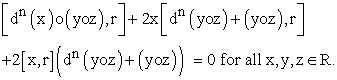(1)

According to our main relation dn(xoy)+(xoy) ∈Z(R), the equation (1), reduces to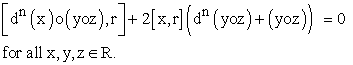(2)

Replacing x by (yoz) in (2),we get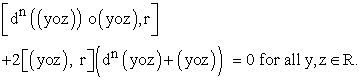(3)

Form the main relation dn(xoy)+(xoy)∈Z(R) for all x,y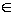R, we obtain [dn(xoy),r]+[xoy,r] =0 for all x,y,r∈R, the replacing r by (xoy),we obtain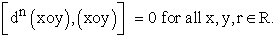(4)

Then substituting (4) in (3), we get 2[dn(yoz)(yoz),r] +[yoz,r] (dn(yoz)+(yoz)) =0 for all z,y,r ∈R.

Then 2dn(yoz)(yoz)r-2rdn(yoz)(yoz)+(yoz)rdn(yoz)-r(yoz)rdn(yoz) +[yoz,r] (yoz)=0 for all z,y,r∈R. Then by using (4) in above equation, we get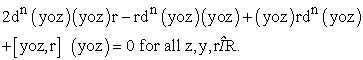(5)

Replacing r by (yoz) with using (4) in (5), we arrive at 2dn(yoz)(yoz)2=0 for all y,z∈R. Since R is 2-torsion free semiprime, we get dn(yoz)(yoz)2=0 for all y,z∈R.

Rirht-multiplying by [x,r], we obtain dn(yoz)(yoz)2[x,r]=0 for all x,y,z,r∈R.

According to Lemma 2, the there exists an ideal U of R such that dn(yoz)(yoz)2∈U⊂ Z (R) for all y,z∈R. This fact lead to have a central ideal U of R, which implies that [x,y]=0 for all x∈U, y∈R.

Apply d to both sided, we obtain [d(x),y]+[x,d(y)]=0 for all x∈U, y∈R. Since U is central ideal, we get [d(x),y]=0 for all x∈U, y∈R. Thus, we get that d is commuting of R, which implies [z,d(z)]=0 for all z∈R. Linearizing [d(z), z] = 0, we obtain [d(z),y] = [z, d(y)]. Hence, we see that the mapping (z, y) → [d(z), y] is a biderivation. By Lemma 4, there exist an idempotent ε ∈C and an element µ∈C such that the algebra (1 - ε)R is commutative (hence, (1 - ε)R ⊆ C), and ε[d(z), y] = εµ[z,y] holds for all z, y∈R. Thus, εd(z) - µε z commutes with every element in R, so that εd(z) - µɛ z∈C. Now, let εd(z) =µε and define a mapping ζ by ζ(z) = (εd(z) - λz) + (1 - ε)d(z). Note that ζ maps in C and that d(z) = λz + ζ(z) holds for every z∈R, by this we complete our proof.

When d=0, we have (xoy)∈Z(R) for all x,y∈R, replacing y by d(x),we obtain d(x2)∈Z(R) for all x∈R, then [x,d(x2)]=0 for all x∈R. According to Lemma 2, then there exists a central ideal U of R, so by same technique, we completes the proof of the theorem.

Corollary 3.2.

Let R be a 2-torsion free semiprime ring and d: R → R be a non-zero derivation on R such that dn(xoy)±(xoy) Z(R) for all x,y∈R, then [dn(xoy),(xoy)n ]=0 for all x,y ∈R, where n is a fixed positive integer.

Proof: According to Theorem 3.1, we have the relation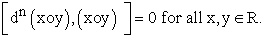(6)

And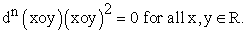(7)

According to (6), we obtain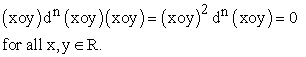(8)

Subtracting (7) and (8),we arrive at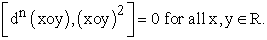(9)

Again right-multiplying of relation (7), by (xoy) gives dn(xoy)(xoy)3=0 for all x,y∈R. Then according to (9) and (6),we arrive at [dn(xoy),(xoy)3 ]=0 for all x,y∈R.

So, if we continue by same technique, arrive to the relation [dn(xoy),(xoy)n]=0 for all x,y∈R, which completes the proof of the corollary.

Corollary 3.3.

Let R be a 2-torsion free semiprime ring and d: R→ R be a non-zero derivation on R such that dn(xoy)±(xoy)∈Z(R) for all x, y ∈R, then R contains a central ideal, where n is a fixed positive integer.

Theorem 3.4.

Let R be a 2-torsion free semiprime ring and d: R→ R be a derivation on R such that dn([x,y])±[x,y]∈Z(R) for all x,y∈R, then there exist C and an additive mapping ζ: R → C such that d(x)=λx+ζ(x) for all x∈R, where n is a fixed positive integer.

Proof: We have the main relation dn([x,y])+[x,y]∈Z(R) for all x,y∈R, now we suppose that d is non-zero derivation on R, then dn([x,y])+[x,y]∈Z(R) for all x,y∈R, then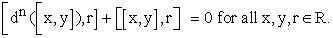(10)

Replacing r by [x,y],gives [dn([x,y]),[x,y]] =0 for all x,y∈R.

Applying Lemma 3, we obtain dn([x,y])∈Z(R) for all x,y∈R.

The substitution this fact in the relation (10), gives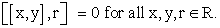(11)

Replacing r by d(z) in (11) with apply Lemma 1, we arrive to d(z)∈Z(R) for all z∈R, which leads to [d(z),z]=0 for all z∈R. Linearizing [d(x), x] = 0 we obtain [d(x), y] = [x, d(y)]. Hence, we see that the mapping (x, y)→[d(x), y] is a biderivation. By Lemma 4, there exist an idempotent ε∈C and an element µ∈C such that the algebra (1 - ε)R is commutative (hence, (1 - ε)R ⊆ C), and ε[d(x), y] = εµ[x,y] holds for all x, y∈R. Thus, εd(x) - µε x commutes with every element in R, so that εd(x) - µε x∈C. Now, let εd(x) =µε and define a mapping ζ by ζ(x) = (εd(x) - λx) + (1 - ε)d(x). Note that ζ maps in C and that d(x) = λx + ζ(x) holds for every x ∈R, by this we complete our proof.

When d=0, we have [x,y]∈Z(R) for all x,y∈R, then by same technique in the first part of proof, we completes the proof.

Theorem 3.5.

Let R be a 2-torsion free semiprime ring and d:R → R be a derivation on R such that dn(xoy)±[x,y]∈Z(R) for all x,y∈R, then there exist C and an additive mapping ζ: R → C such that d(x)=λx+ζ(x) for all x∈R, where n is a fixed positive integer.

Proof: We have the relation dn(xoy)+[x,y]∈Z(R) for all x,y∈R.

Now, when d is non-zero derivation on R, we have the relation dn(xoy)+[x,y]∈Z(R) for all x,y∈R. Then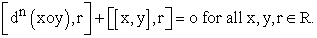(12)

Replacing r by [x,y], we obtain [dn(xoy),[x,y]]=o for all x,y∈R. Applying Lemma 3, we obtain dn(xoy)∈Z(R) for all x,y∈R, thus by using this fact in (12), we arrive to [[x,y],r]=o for all x,y,r∈R.

Replaicing r by d(z) with applying Lemma 1, we obtain [d(z),z]=0 for all z∈R. Then Linearizing [d(z), z] = 0 we obtain [d(x), y] = [x, d(y)]. Hence, we see that the mapping (x, y)→[d(x), y] is a biderivation. By Lemma 4, there exist an idempotent ε∈C and an element µ∈C such that the algebra (1 - ε)R is commutative (hence, (1 - ε)R ⊆ C), and ε[d(x), y] = εµ[x,y] holds for all x, y∈R. Thus, εd(x) - µε x commutes with every element in R, so that εd(x) - µε x∈C. Now, let εd(x) =µε and define a mapping ζ by ζ(x) = (εd(x) - λx) + (1 - ε)d(x). Note that ζ maps in C and that d(x) = λx + ζ(x) holds for every xR, by this we complete our proof.

When d=0, we have [x,y]∈Z(R) for all x,y∈R, then by same technique in the first part of proof of Theorem 3.4, we completes the proof.

Theorem 3.6.

Let R be a 2-torsion free semiprime ring and U is a non-zero ideal of R, d: R → R be a derivation on R. If R admits d to satisfy dn([x,y])±(xoy)∈Z(R) for all x,y∈U, then there exist C and an additive mapping ζ: R→ C such that d(x)=λx+ζ(x) for all x∈R, where n is a fixed positive integer.

Proof: In our theorem, we have the main relation dn([x,y])+(xoy)∈Z(R) for all x,y∈U. Now, we assume that d is non-zero derivation of R, then dn([x,y])+(xoy)∈Z(R) for all x,y∈U, which gives [dn([x,y]),r]+[xoy,r]=0 for all x,y∈U.

Replacing x by y and r by d(y),we obtain 2[d(y),y2]=0 for all y∈U. Since R is 2-torsion free semiprime with apply

Lemma 5, we have R contains a non-zero central ideal U, then for all x∈U, there exists y∈R, such that [x,y]=0 for all x∈U, y∈R.

Apply d to both sided, we obtain [d(x),y]+[x,d(y)]=0 for all x∈U,y∈R. Since U is central ideal, we get [d(x),y]=0 for all x∈U, y∈R. Thus, we get that d is commuting of R, which implies [z,d(z)]=0 for all z∈R.

Linearizing [d(z), z] = 0, we obtain [d(z),y] = [z, d(y)]. Hence, we see that the mapping (z, y)→[d(z), y] is a biderivation. By Lemma 4, there exist an idempotent ε ∈C and an element µ ∈C such that the algebra (1 - ε)R is commutative (hence, (1 - ε)R ⊆ C), and ε[d(z), y] = εµ[z,y] holds for all z, y ∈ R. Thus, εd(z) - µε z commutes with every element in R, so that εd(z) - µε z∈C. Now, let εd(z) =µε and define a mapping ζ by ζ(z) = (εd(z) - λz) + (1 - ε)d(z). Note that ζ maps in C and that d(z) = λz + ζ(z) holds for every z∈R, by this we complete our proof. When d=0, we have (xoy)∈Z(R) for all x,y∈R, the we completes the proof of the theorem by same method in part second of Theorem 3.1.

Corollary 3.7.

Let R be a 2-torsion free semiprime ring and d: R → R be a derivation on R. If R admits d to satisfy dn([x,y])±(xoy) ∈Z(R) for all x,y∈ R, then R contains anon-zero central ideal, where n is a fixed positive integer.

Theorem 3.8.

Let R be a 2-torsion free semiprime ring and d: R→ R be a derivation on R. If R admits d to satisfy dn(xoy)±dq(xoy)±[x,y]Z(R) for all x,yR, then there exist C and an additive mapping ζ: R→ C such that d(x)=λx+ζ(x) for all x∈R, where n and q be a fixed positive integers.

Proof: We have the relation dn(xoy)+dq(xoy)+[x,y] ∈Z(R) for all x,y∈R. Suppose that d is non-zero derivation of R, we have dn(xoy)+dq(xoy)+[x,y] ∈Z(R) for all x,y∈R, then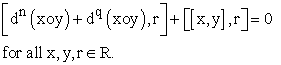(13)

Replacing r by [x,y], we obtain [dn(xoy)+dq(xoy), [x,y]]=0 for all x,y∈R. Apply Lemma1, and substituting that result in relation (13), we get [[x,y],r]=0 for all x,y,r∈R. Then replacing r by d(z), we get [[x,y],d(z)]=0 for all x,y,z∈R. Then by apply Lemma1, we arrive to [d(z),z]=0 for all z ∈R. Linearizing [d(x), x] = 0 we obtain [d(x), y] = [x, d(y)]. Hence, we see that the mapping (x, y)→[d(x), y] is a biderivation. By Lemma 4, there exist an idempotent ε ∈C and an element µ ∈C such that the algebra (1 - ε)R is commutative (hence, (1 - ε)R ⊆ C), and ε[d(x), y] = εµ[x,y] holds for all x, y ∈ R. Thus, εd(x) - µε x commutes with every element in R, so that εd(x) - µε x ∈C. Now, let εd(x) =µε and define a mapping ζ by ζ(x) = (εd(x) - λx) + (1 - ε)d(x). Note that ζ maps in C and that d(x) = λx + ζ(x) holds for every x ∈R, by this we complete our proof.

When d=0, we get the relation [x,y]∈Z(R) for all x,y∈R. Therefore, by using same method in the first part of proof of the theorem, we completes our proof.

Proceeding on the same line with necessary variations, we can prove the following theorem.

Theorem 3.9.

Let R be a 2-torsion free semiprime ring and d: R→ R be a derivation on R. If R admits d to satisfy

(i) dn(xoy)±dq([x,y])±[x,y]∈Z(R) for all x,y∈R.

(ii)dn([x,y])±dq([x,y])±[x,y]∈Z(R) for all x,y∈R.

then there exist C and an additive mapping ζ: R → C such that d(x)=λx+ζ(x) for all x∈R, where n and q be a fixed positive integers.

Theorem 3.10.

Let R be a 2-torsion free semiprime ring and d: R→ R be a derivation on R. If R admits d to satisfy dn([x,y])±dq([x,y])±(xoy) ∈Z(R) for all x,y∈R, then there exist C and an additive mapping ζ: R →C such that d(x)=λx+ζ(x) for all x ∈R, where n and q be a fixed positive integers.

Proof: The main relation it is dn([x,y])+dq([x,y])+(xoy) ∈Z(R) for all x,y∈ R. Suppose that d is non-zero derivation of R, we have dn([x,y])+dq([x,y])+(xoy)∈Z(R) for all x,y∈ R, then [dn([x,y])+dq([x,y]),r]+[xoy,r]=0 for all x,y∈R

Replacing x by y, gives 2[y2,r]=0 for all y,r∈R. Since R is 2-torsion free semiprime, we obtain y2∈Z(R) with apply Lemma 3, we arrive to [y,r]=[y,d(y)]=0 for all y,r ∈R.

When d=0, the our main relation reduces to (xoy)∈Z(R) for all x,y ∈R, replacing y by x, with using R is 2-torsion free semiprime, we get

[x2,r]=0 for all x,r ∈R. This relation gives x2∈Z (R),with apply Lemma 3, we arrive to

[x,r]=[x,d(x)]=0 for all x,r ∈R. Linearizing [d(x), x] = 0 we obtain [d(x), y] = [x, d(y)]. Hence, we see that the mapping (x, y) → [d(x), y] is a biderivation. By Lemma 4, there exist an idempotent ε∈C and an element µ ∈C such that the algebra (1 - ε)R is commutative (hence, (1 - ε)R ⊆ C), and ε[d(x), y] = εµ[x,y] holds for all x, y ∈ R. Thus, εd(x) - µε x commutes with every element in R, so that εd(x) - µε x∈C. Now, let εd(x) =µε and define a mapping ζ by ζ(x) = (εd(x) - λx) + (1 - ε)d(x). Note that ζ maps in C and that d(x) = λx + ζ(x) holds for every x ∈R, by this we complete our proof.

When d=0, we have (xoy) ∈ Z(R) for all x,y∈ R, the we completes the proof of the theorem by same method in part second of Theorem 3.1.

Theorem 3.11.

Let R be a 2-torsion free semiprime ring and d:R→ R be a derivation on R. If R admits a derivation d to satisfy dn(xoy)±dq(xoy)±(xoy)∈Z(R) for all x,y∈ R, then there exist C and an additive mapping ζ: R → C such that d(x)=λx+ζ(x) for all x∈ R, where n and q be a fixed positive integer.

Proof: We have the relation dn(xoy)+dq(xoy)+(xoy) ∈Z(R) for all x,y∈ R, which can be written in the form gw(xoy)+(xoy)∈Z(R) for all x,y∈ R, where g(xoy) stands for dn(xoy)+dq(xoy) and w stands for a fixed positive integer n and q.

Then, we have [gw(xoy)+(xoy),r]=0 for all x,y∈ R. Thus by same technique in the proof of the Theorem 3.1, we completes the proof.

Corollary 3.12.

Let R be a 2-torsion free semiprime ring and d: R→ R be a non-zero derivation on R such that dn(xoy) ±dq(xoy)±(xoy)∈Z(R) for all x,y ∈R then [dn(xoy) +dq(xoy),(xoy)n]=0 for all x,y∈ R, where n and q be a fixed positive integers.

Corollary 3.13.

Let R be a 2-torsion free semiprime ring and d:R→ R be a non-zero derivation on R such that dn(xoy) ±dq(xoy)±(xoy) ∈ Z(R) for all x,y ∈R, then R contains a central ideal, where n and q be a fixed positive integers.

Remark 2.13.

In our theorems we cannot exclude the condition torsion free, the following example explain that.

Example 2.14.

We denote by Z the integer system, let: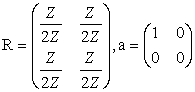and d is the inner derivation induced by a (every derivation is inner derivation), that is, d(x) [a, x], for all x R. Then R is a non-commutative prime ring with char R2, and d([x,y])[x,y] Z(R), for all x, y R.

Then by similar approach we can show otherwise.

### Acknowledgements

The authors are greatly indebted to the referee for his careful reading the paper.

### References

  S. Andima and H. Pajoohesh, Commutativity of prime rings with derivations, Acta Math. Hungarica, Vol. 128 (1-2) (2010), 1-14.In article CrossRef  G.A. Baker, A new derivation of Newton’s identities and their application to the calculation of the eigenvalues of a matrix, J. Soc. Indust. Appl. Math. 7 (1959) 143-148.In article CrossRef  H. E. Bell and M. N. Daif, On derivations and commutativity in prime rings. Acta Math. Hungar. Vol. 66 (1995), 337-343.In article CrossRef  I. N. Herstein, A note on derivations, Canad. Math. Bull, 21 (1978), 369-370.In article CrossRef  da Providencia, Joao, The numerical ranges of derivations and quantum physics, Special Issue: The numerical range and numerical radius. Linear and Multilinear Algebra 37 (1994), no. 1-3, 213-220.In article  H. Pajoohesh, Positive derivations on lattice ordered rings of Matrices, Quaestiones Mathematicae, Vol. 30, (2007), 275-284.In article CrossRef  E. C. Posner, Derivations in prime rings, Proc. Amer. Math. Soc. 8 (1957), 1093-1100.In article CrossRef  McCoy, Neal.H, Theory of Rings, Mac Millan &Co. (1970).In article  Matej Bresar, Centralizing Mappings on Von Neumann Algebras, Proceedings of the American Mathematical Society, Vol. 120, Number 3, (1994), 709-713.In article  N. Divinsky, On commuting automorphisms of rings, Trans. Roy. Soc. Canad. Sect. III. (3) 49 (1955), 19-22.In article  Muhammad A.C. and S.,S. Mohammed, Generalized inverses of centralizer of semiprime rings, Aequations Mathematicae, 71 (2006), 1-7.In article CrossRef  Muhammad A. and A.B. Thaheem, A note on a pair of derivations of semiprime rings, IJMMS, 39 (2004), 2097-2102.In article  B.Zalar, On centralizers of semiprime rings, Comment. Math. Univ. Carolinae, 32 (4) (1991), 609-614.In article  Q.Deng and H.E.Bell, On derivations and commutativity in semiprime rings, Comm. Algebra 23 (1995), 3705-3713.In article CrossRef  S. A. Amitsur, On rings of quotients, Sympos. Math. 8 (1972), 149-164.In article  Matej Bresar, On certain pairs of functions of semiprime rings, Proceedings of the American Mathematical Society, Vol. 120, Number 3, March (1994), 709-713.In article CrossRef  I.N. Herstein, Rings with involution, University of Chicago Press, Chicago, Illinois, (1976).In article  Mehsin Jabel, Generalized derivations of semiprime rings, Lambert Academic Publishing, Germany (2012).In article  Mehsin Jabel, Commutativity results with derivations on semiprime rings, Journal of Mathematical and Computational Science, London-UK, No. 4, 2 (2012), 853-865.In article  Mehsin Jabel, Derivations of semiprime rings with left cancellation property, Cayley Journal of Mathematics, Vol. 1, (1) (2012), 71-75.In article  C.Lanski, An enel condition with derivation for left ideals, Proc. Amer. Math. Soc., 125 (2) (1997), 339-345.In article CrossRef